﻿ 一种基于Multi-Egocentric视频运动轨迹重建的多目标跟踪算法
«上一篇文章快速检索 高级检索

 智能系统学报2019, Vol. 14Issue (2): 246-253  DOI: 10.11992/tis.2017090030

### 引用本文OU Weiqi, YIN Hui, XU Hongli, et al. A multi-object tracking algorithm based on trajectory reconstruction on multi-egocentric video[J]. CAAI Transactions on Intelligent Systems, 2019, 14(2): 246-253. DOI: 10.11992/tis.201709003.### 文章历史

1. 北京交通大学 计算机与信息技术学院，北京 100044;
2. 北京交通大学 交通数据分析与挖掘北京市重点实验室，北京 100044

A multi-object tracking algorithm based on trajectory reconstruction on multi-egocentric video
OU Weiqi 1,2, YIN Hui 1,2, XU Hongli 1,2, LIU Zhihao 1,2
1. Department of Computer and Information Technology, Beijing Jiaotong University, Beijing 100044, China;
2. Beijing Key Lab of Transportation Data Analysis and Mining, Beijing Jiaotong University, Beijing 100044, China
Abstract: In egocentric video, objects have the characteristics of violent motion, frequent occlusion, so it brings much trouble to carrying out the tracking task. In this paper, we propose a multi-object tracking algorithm based on the motion trajectory reconstruction of multi-egocentric video from different visual angles egocentric videos. First, this method is based on the homographic constraint of multi-view synch frames to fix position of occluded and missing object. Second, using the relative position constraint relation of multi-angle target, the trajectory is reconstructed to locate the target position. Meanwhile, the trajectory of the object is optimized by constructing the motion model of object. Then the continuous trajectory of the object is obtained and the problem of the discontinuity trajectory in multi-object tracking is resolved. In the end, the performance of proposed method has been verified by using the multi-view video dataset which is created by us.
Key words: Multi-Egocentric video    trajectory reconstruction    multi-object tracking    homographic constraint    epipolar geometry constraint    spatial reconstruction    Kalman filter    motion model

1 基于运动轨迹重建的多目标跟踪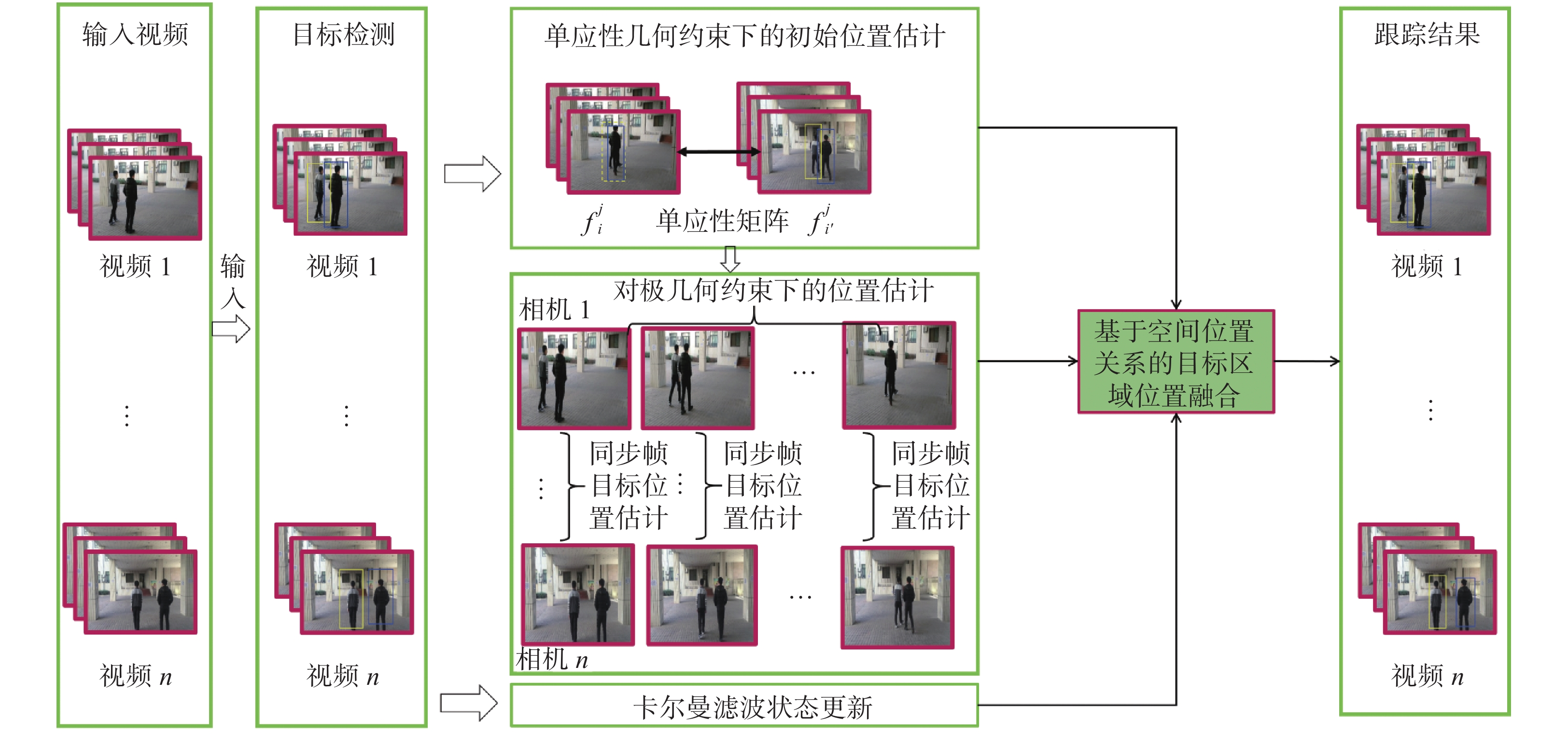Download: 图 1 基于运动轨迹重建的多目标跟踪算法流程 Fig. 1 Flow chart of multi-target tracking algorithm based on trajectory reconstruction

1.1 多视角辅助下的目标初始位置估计

$f_i^j$ 为视角 $i$ ( $i$ =1,2,···,n)第 $j$ 帧图像，其中包含 $m$ 个目标 ${P_k},\text{其中}k = 1,2,\cdots ,m$ $B_i^j$ 表示目标检测在 $f_i^j$ 的检测框集合， $B_i^j = \{ b_{ik}^j|k = 1,2,\cdots,m\}$ $b_{ik}^j =\{ x_{ik}^j,y_{ik}^j,$ $\tilde x_{ik}^j,\tilde y_{ik}^j\}$ 表示第 $k$ 个目标的Bounding Box，其中 $x_{ik}^j{\text、}y_{ik}^j{\text、}$ $\tilde x_{ik}^j{\text、}\tilde y_{ik}^j$ 分别表示 $b_{ik}^j$ 的左上角横坐标、左上角纵坐标、右下角横坐标、右下角纵坐标，设 $t_{ik}^j$ 作为检测结果中目标 $k$ 在视角 $i$ $j$ 帧的轨迹点：

 $t_{ik}^j = [\tilde x_{ik}^j,(y_{ik}^j{\rm{ + }}\tilde y_{ik}^j)/2]$ (1)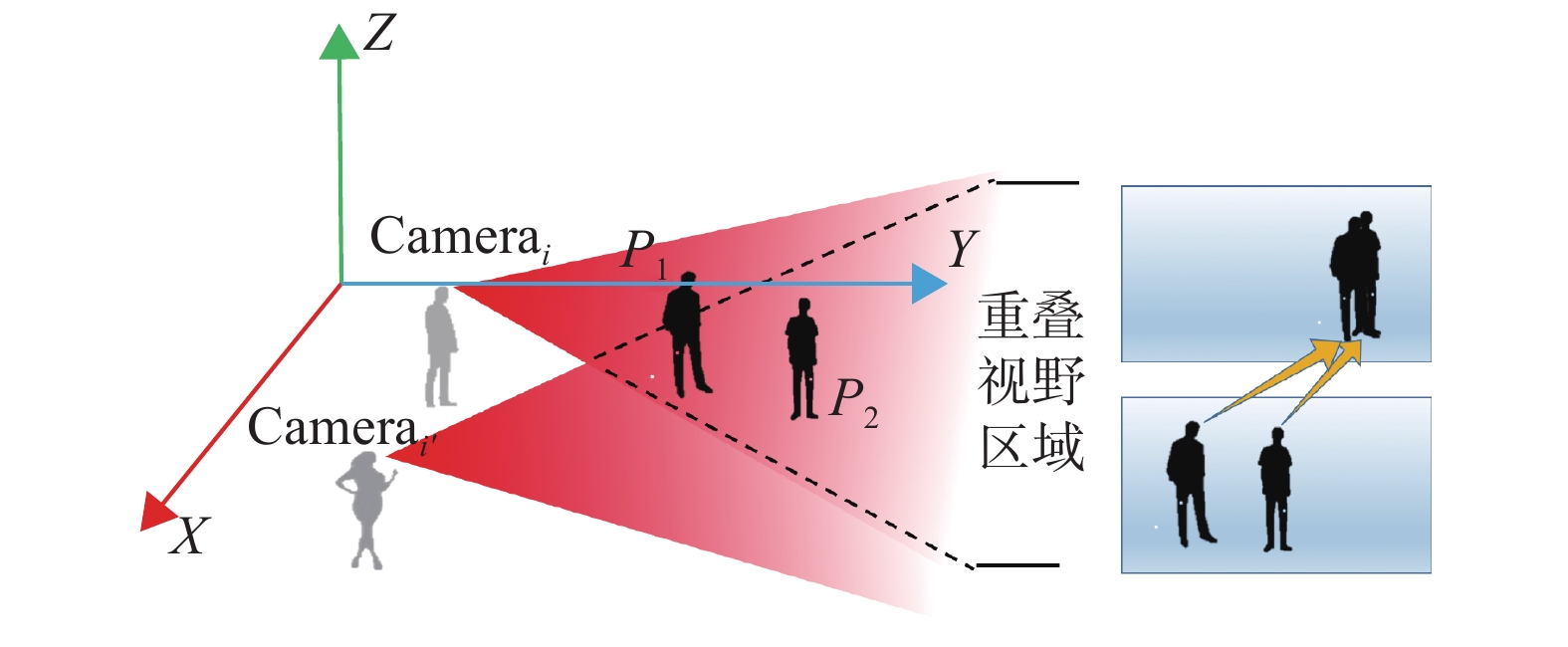Download: 图 2 多视角中目标之间相互遮挡示意图 Fig. 2 Multi-view of the occlusion between targets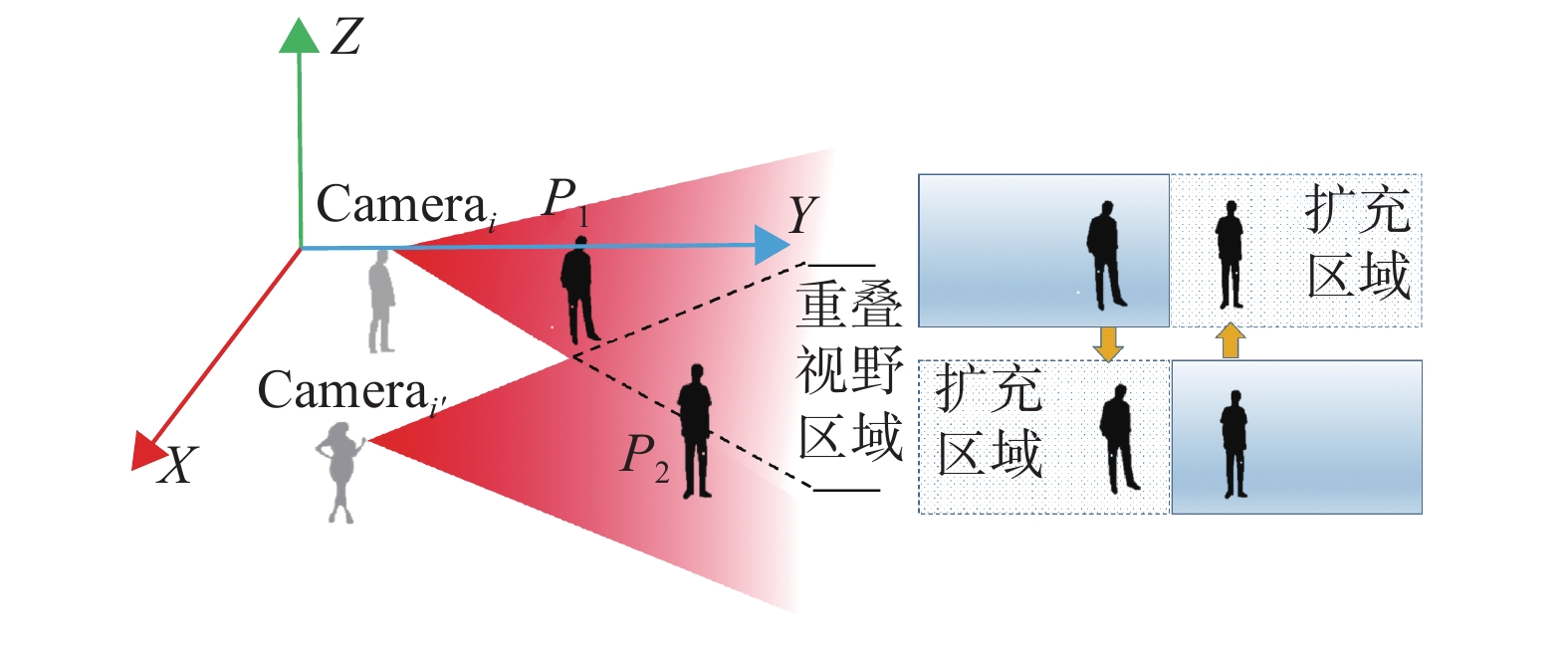Download: 图 3 多视角移动造成目标丢失示意图 Fig. 3 Multi-view movement causes the target to lose the sketch map

2) $f_{i'}^j$ 的目标所在位置 $t_{i'k}^j$

3) 采用SIFT角点检测算法对 $f_i^j$ $f_{i'}^j$ 两帧提取地面特征点，得到匹配点集合 ${ P}_i^j$ ${ P}_{i'}^j$

4) 利用匹配点构建方程(2)，并利用RANSAC算法剔除误匹配点求解单应性矩阵；

 ${ P}_i^j = {{HP}}_{i'}^j$ (2)

5)根据式(2)把 $t_{ik}^j$ 代入求得 $f_{i'}^j$ 中被遮挡目标 $k$ 所在位置 $t_{{i'}k}^j$

1.2 多视角轨迹重建位置估计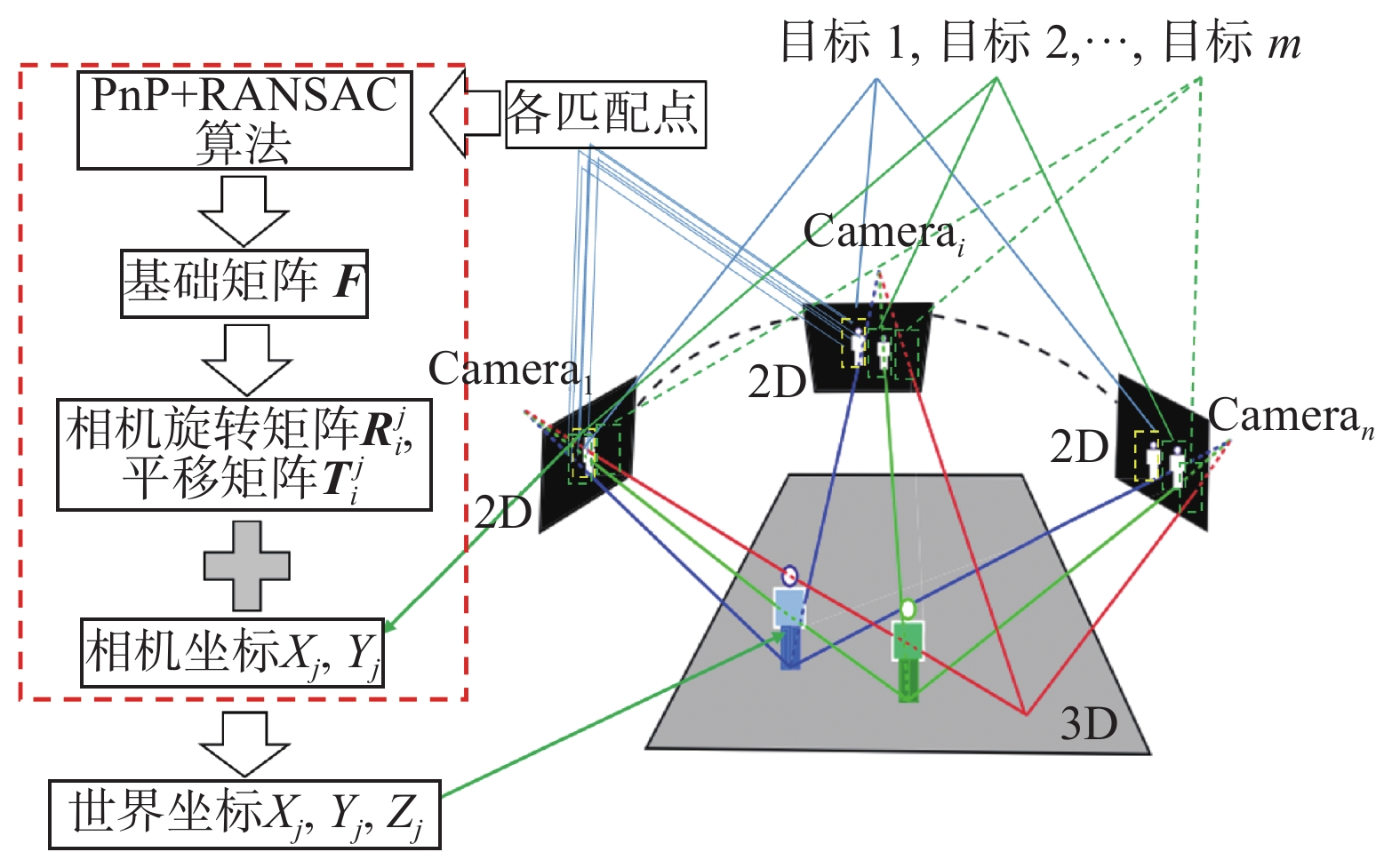Download: 图 4 同步帧目标位置估计算法图 Fig. 4 Sketch map of synchronous frame target location estimation algorithm
 ${({ P}_i^j)^{\rm{T}} }{{F}}_{i,i'}^j{ P}_{i'}^j = {{0}}$ (3)
 ${{E}}_{i,i'}^j = {({{ K}_i})^{\rm{T}} }{{F}}_{i,i'}^j{{ K}_{i'}}$ (4)

1.3 基于轨迹重建的多目标跟踪

 $\hat u_k^{j/j - 1} = {{A}}u_k^{j - 1} + {{B}}c_k^{j - 1} + w_k^{j - 1}$ (5)

 ${{A}} = \left[ {\begin{array}{*{20}{c}} 1&T&0&0 \\ 0&1&0&0 \\ 0&0&1&T \\ 0&0&0&1 \end{array}} \right]$

 ${{B}} = \left[ {\begin{array}{*{20}{c}} 1&0&0&0 \\ 0&0&1&0 \end{array}} \right]$

 $u_k^j = \hat u_k^{j/j - 1} + K_k^j[z_k^j - {{H}}\hat u_k^{j/j - 1}]$ (6)

1.4 基于空间位置关系的目标区域位置融合

 \left\{ \begin{aligned} & O_k^j = {\omega _1}t_k^j + {\omega _2}s_k^j + {\omega _3}u_k^j \\ & {\omega _1} + {\omega _2} + {\omega _3} = 1 \end{aligned} \right. (7)

2 实验结果与分析表 1 实验采用的数据集 Tab.1 Experimental data sets information

 ${\rm Err} = \sqrt {{{({r_t}.x - {r_g}.x)}^2} + {{({r_t}.y - {r_g}.y)}^2}}$ (8)

 ${\rm S}{\rm{core}} = \frac{{{\rm area}({r_t} \cap {r_g})}}{{{\rm area}({r_t} \cup {r_g})}}$ (9)
2.1 在BJMOT数据集上的实验结果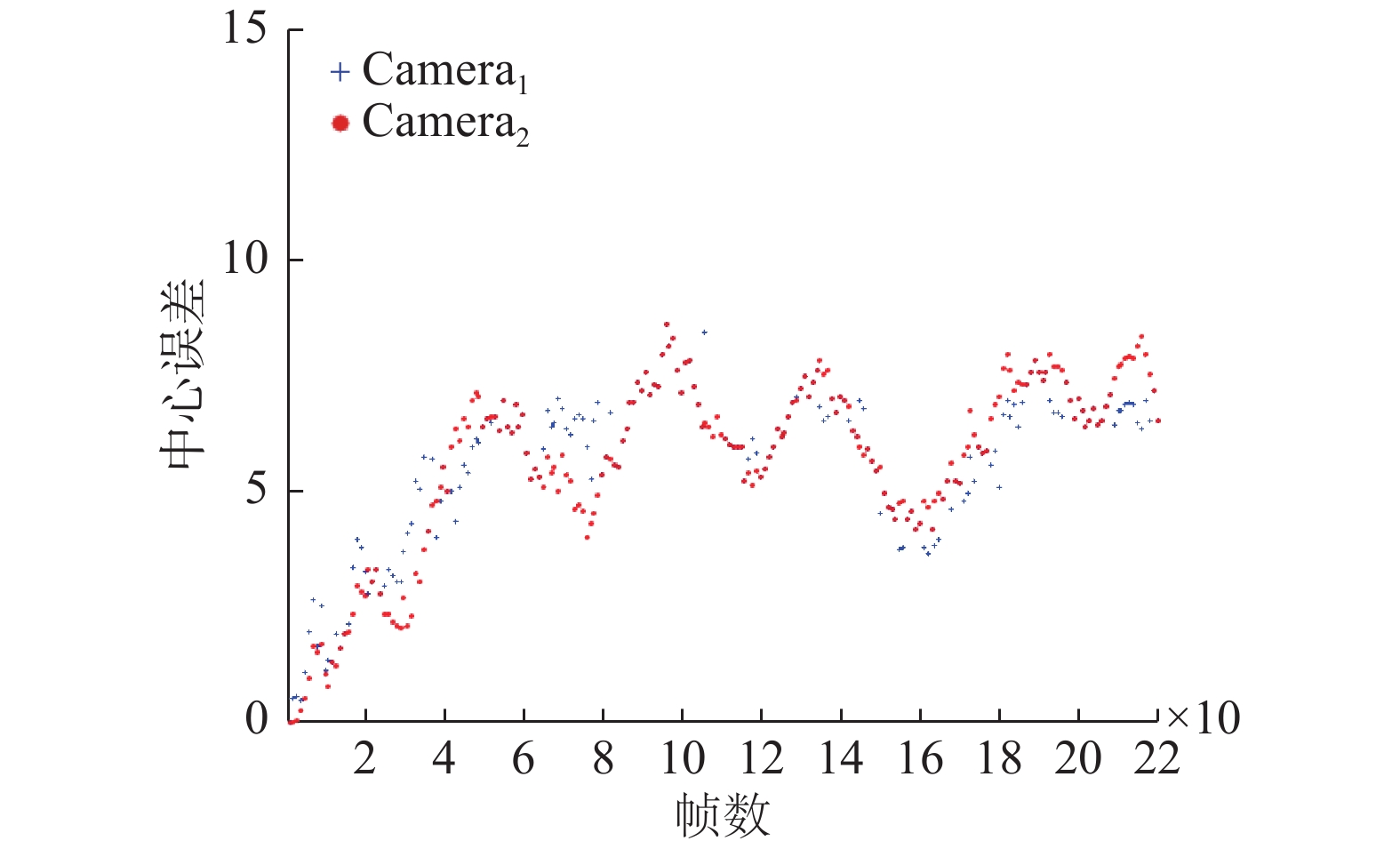Download: 图 5 本文算法在BJMOT数据集的中心误差曲线 Fig. 5 The central error curve of the algorithm in BJMOT dataset表 2 本文算法在BJMOT上的平均中心误差和平均重叠率 Tab.2 The mean center error and the average overlap rate of the proposed algorithm over BJMOTDownload: 图 6 本文算法在BJMOT数据集第1个视角视频中的分步实验结果 Fig. 6 The experimental results of ours algorithm in the first video sequences of the BJMOT datasetsDownload: 图 7 本文算法在BJMOT数据集第2个视角视频中的分步实验结果 Fig. 7 The experimental results of ours algorithm in the in the second video sequences of BJMOT datasets
2.2 在EPLF-campus4数据集上的实验结果表 3 3个跟踪算法在EPLF-campus4上的平均重叠率 Tab.3 The average overlap rate of 3 tracking algorithms on EPLF-campus4表 4 3个跟踪算法在EPLF-campus4上的平均中心误差 Tab.4 Average center error of 3 tracking algorithms on EPLF-campus4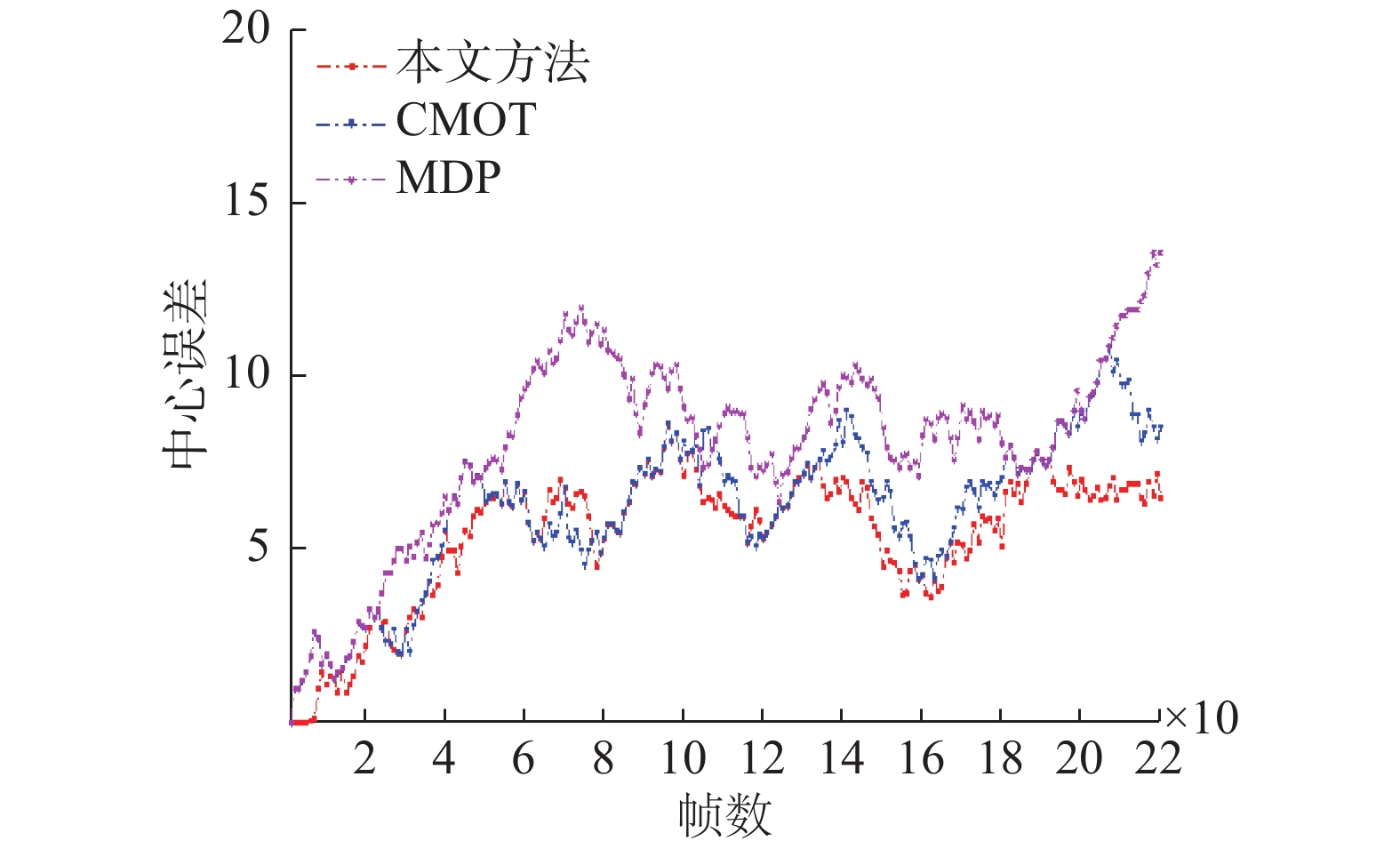Download: 图 8 3种算法在Camera1视频中的中心误差曲线 Fig. 8 The center error of three tracking algorithms on camera1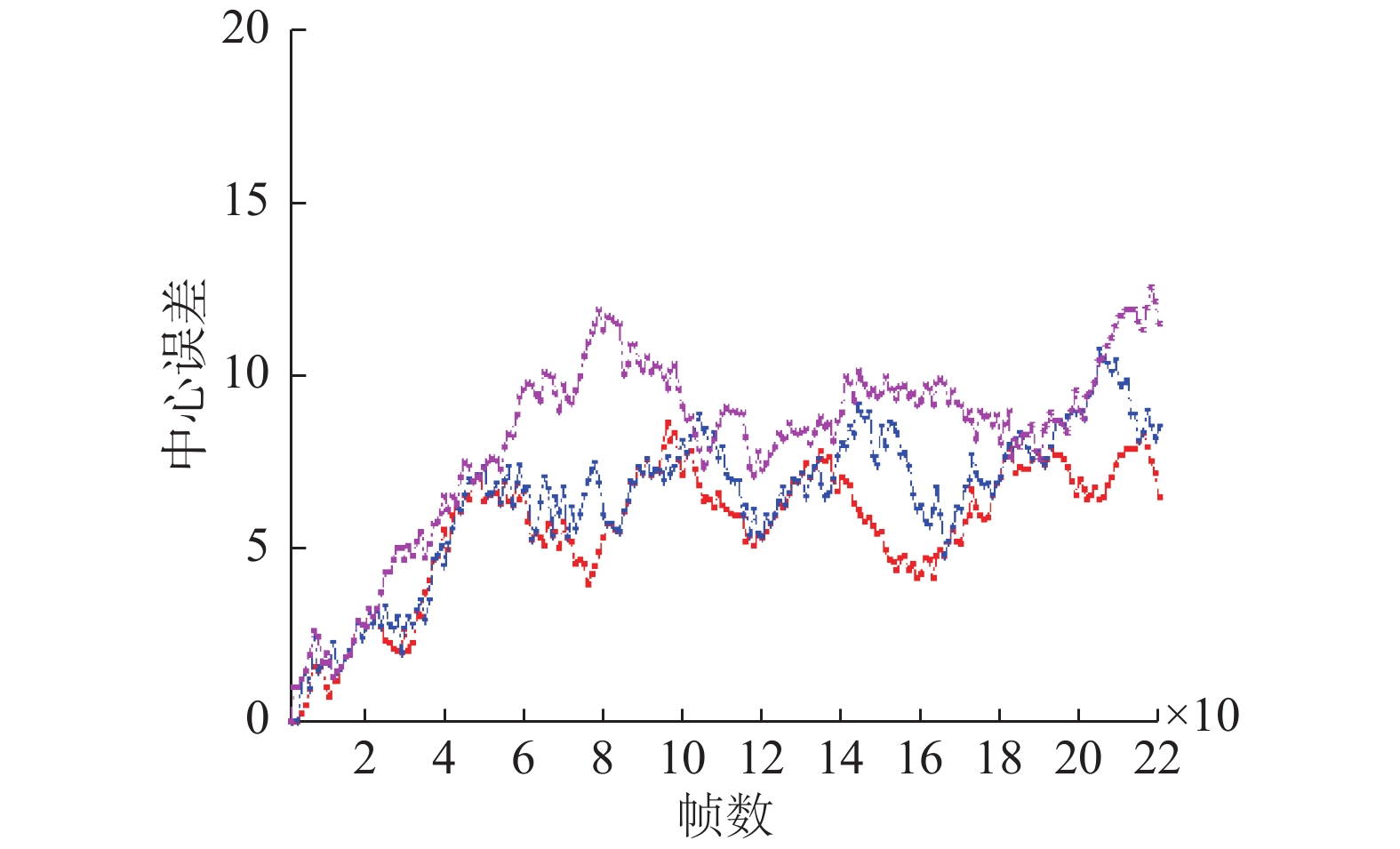Download: 图 9 3种算法在Camera2视频中的中心误差曲线 Fig. 9 The center error of three tracking algorithms on camera2Download: 图 10 3种算法在EPLF-campus4数据集第一个视角视频中的跟踪对比结果 Fig. 10 Tracking results of three algorithms in the first video of EPLF-campus4 datasetsDownload: 图 11 3种算法在EPLF-campus4数据集第二个视角视频中的跟踪对比结果 Fig. 11 Tracking results of three algorithms in the second video of EPLF-campus4 datasets
3 结束语

  SHAN Caifeng, WEI Yucheng, TAN Tieniu, et al. Real time hand tracking by combining particle filtering and mean shift[C]//Proceedings of the 6th IEEE International Conference on Automatic Face and Gesture Recognition. Seoul, South Korea, 2004: 669–674. (0)  ZHANG Lei, LI Yuan, NEVATIA R. Global data association for multi-object tracking using network flows[C]//Proceedings of 2008 IEEE Conference on Computer Vision and Pattern Recognition. Anchorage, AK, USA, 2008: 1–8. (0)  AVIDAN S. Ensemble tracking[J]. IEEE transactions on pattern analysis and machine intelligence, 2007, 29(2): 261-271. DOI:10.1109/TPAMI.2007.35 (0)  XU Yuanlu, LIU Xiaobai, LIU Yang, et al. Multi-view people tracking via hierarchical trajectory composition[C]//Proceedings of 2016 IEEE Conference on Computer Vision and Pattern Recognition. Las Vegas, NV, USA, 2016: 4256–4265. (0)  HE Shengfeng, YANG Qingxiong, LAU R W H, et al. Visual tracking via locality sensitive histograms[C]//Proceedings of 2013 IEEE Conference on Computer Vision and Pattern Recognition. Portland, USA, 2013: 2427–2434. (0)  FLEURET F, BERCLAZ J, LENGAGNE R, et al. Multicamera people tracking with a probabilistic occupancy map[J]. IEEE transactions on pattern analysis and machine intelligence, 2008, 30(2): 267-282. DOI:10.1109/TPAMI.2007.1174 (0)  MEI Xue, LING Haibo. Robust visual tracking using ℓ1 minimization[C]//Proceedings of the 12th IEEE International Conference on Computer Vision. Kyoto, Japan, 2009: 1436–1443. (0)  BABENKO B, YANG M H, BELONGIE S. Robust object tracking with online multiple instance learning[J]. IEEE transactions on pattern analysis and machine intelligence, 2011, 33(8): 1619-1632. DOI:10.1109/TPAMI.2010.226 (0)  王宇霞, 赵清杰, 蔡艺明, 等. 基于自重构粒子滤波算法的目标跟踪[J]. 计算机学报, 2016, 39(7): 1294-1306. WANG Yuxia, ZHAO Qingjie, CAI Yiming, et al. Tracking by auto-reconstructing particle filter trackers[J]. Chinese journal of computers, 2016, 39(7): 1294-1306. (0)  BAE S H, YOON K J. Robust online multi-object tracking based on tracklet confidence and online discriminative appearance learning[C]//Proceedings of 2014 IEEE Conference on Computer Vision and Pattern Recognition. Columbus, OH, USA, 2014: 1218–1225. (0)  DICLE C, CAMPS O I, SZNAIER M. The way they move: tracking multiple targets with similar appearance[C]//Proceedings of 2013 IEEE International Conference on Computer Vision. Sydney, NSW, Australia, 2013: 2304–2311. (0)  XIANG Yu, ALAHI A, SAVARESE S. Learning to track: online multi-object tracking by decision making[C]//Proceedings of 2015 IEEE International Conference on Computer Vision. Santiago, Chile, 2015: 4705–4713. (0)  NAM H, HAN B. Learning multi-domain convolutional neural networks for visual tracking[C]//Proceedings of 2016 IEEE Conference on Computer Vision and Pattern Recognition. Las Vegas, NV, USA, 2016: 4293–4302. (0)  KHAN S M, YAN Pingkun, SHAH M. A homographic framework for the fusion of multi-view silhouettes[C]//Proceedings of the 11th International Conference on Computer Vision. Rio de Janeiro, Brazil, 2007: 1–8. (0)  FISCHLER M A, BOLLES R C. Random sample consensus: a paradigm for model fitting with applications to image analysis and automated cartography[J]. Communications of the ACM, 1981, 24(6): 381-395. DOI:10.1145/358669.358692 (0)  ZHANG Z. A flexible new technique for camera calibration[J]. IEEE transactions on pattern analysis and machine intelligence, 2000, 22(11): 1330-1334. DOI:10.1109/34.888718 (0)  SCHWEIGHOFER G, PINZ A. Robust pose estimation from a planar target[J]. IEEE transactions on pattern analysis and machine intelligence, 2006, 28(12): 2024-2030. DOI:10.1109/TPAMI.2006.252 (0)  BEKIR E. Adaptive Kalman filter for tracking maneuvering targets[J]. Journal of guidance, control, and dynamics, 2015, 6(5): 414-416. (0)  DOLLÁR P, APPEL R, BELONGIE S, et al. Fast feature pyramids for object detection[J]. IEEE transactions on pattern analysis and machine intelligence, 2014, 36(8): 1532-1545. DOI:10.1109/TPAMI.2014.2300479 (0)  BERNARDI K, STIEFELHAGEN R. Evaluating multiple object tracking performance: the CLEAR MOT metrics[J]. EURASIP Journal on image and video processing, 2008, 2008(1): 246309. (0)